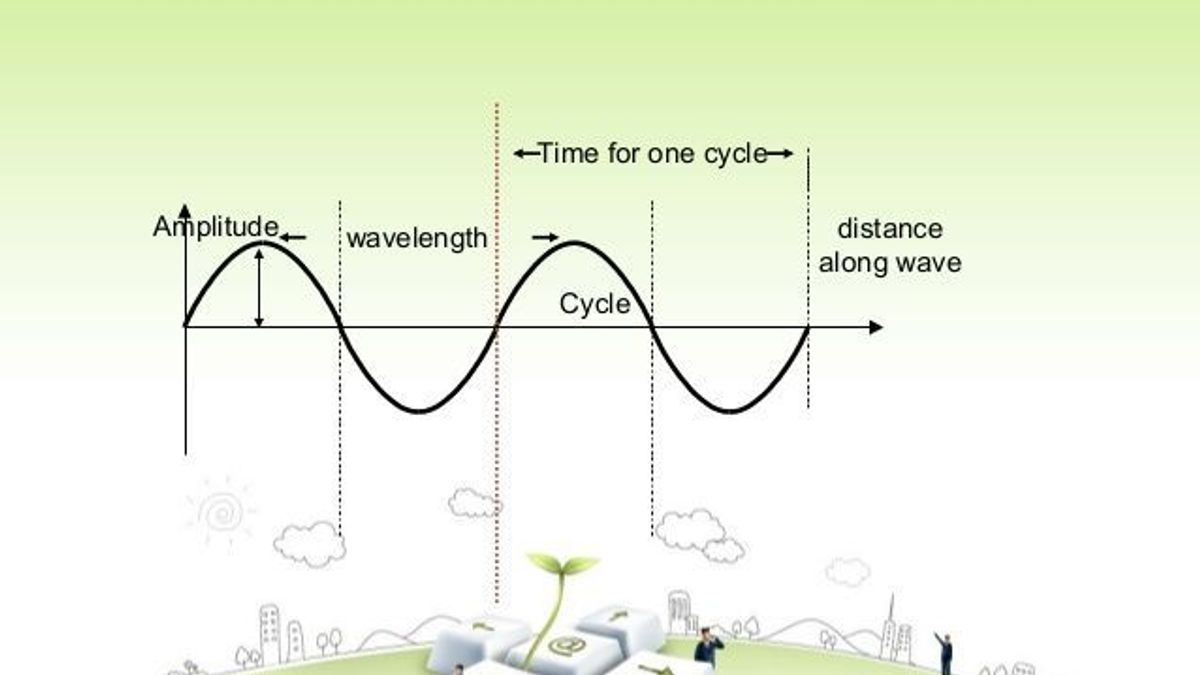# What are the characteristics of Sound Waves?

Sound is a form of energy which makes us hear. It travels in the form of wave. Sound wave can be described by five characteristics. Let us study through this article about it.What are the characteristics of Sound Waves?

The sensation felt by our ears is called sound. It is a form of energy which makes us hear. We hear several sounds around us in our everyday life.

We know that sound travels in the form of wave.

A wave is a vibratory disturbance in a medium which carries energy from one point to another without there being a direct contact between the two points.

We can say that a wave is produced by the vibrations of the particles of the medium through which it passes.

There are two types of waves: Longitudinal waves and Transverse waves.

Longitudinal Waves: A wave in which the particles of the medium vibrate back and forth in the ‘same direction’ in which the wave is moving.  Medium can be solid, liquid or gases. Therefore, sound waves are longitudinal waves.

Transverse Waves: A wave in which the particles of the medium vibrate up and down ‘at right angles’ to the direction in which the wave is moving. These waves are produced only in a solids and liquids but not in gases.

Sound is a longitudinal wave which consists of compressions and rarefactions travelling through a medium.

Sound wave can be described by five characteristics: Wavelength, Amplitude, Time-Period, Frequency and Velocity or Speed.

1. Wavelength

The minimum distance in which a sound wave repeats itself is called its wavelength. That is it is the length of one complete wave. It is denoted by a Greek letter λ (lambda).  We know that in a sound wave, the combined length of a compression and an adjacent rarefaction is called its wavelength. Also, the distance between the centres of two consecutive compressions or two consecutive rarefactions is equal to its wavelength.

Note: The distance between the centres of a compression and an adjacent rarefaction is equal to half of its wavelength i.e. λ/2. The S.I unit for measuring wavelength is metre (m).

2. Amplitude

When a wave passes through a medium, the particles of the medium get displaced temporarily from their original undisturbed positions. The maximum displacement of the particles of the medium from their original undisturbed positions, when a wave passes through the medium is called amplitude of the wave. In fact the amplitude is used to describe the size of the wave. The S.I unit of measurement of amplitude is metre (m) though sometimes it is also measured in centimetres.  Do you know that the amplitude of a wave is the same as the amplitude of the vibrating body producing the wave?

3. Time-Period

The time required to produce one complete wave or cycle or cycle is called time-period of the wave. Now, one complete wave is produced by one full vibration of the vibrating body. So, we can say that the time taken to complete one vibration is known as time-period. It is denoted by letter T. The unit of measurement of time-period is second (s).

Why Speed and Velocity are not always equal in magnitude?

4. Frequency

Source: www.media.openschool.com

The number of complete waves or cycles produced in one second is called frequency of the wave. Since one complete wave is produced by one full vibration of the vibrating body, so we can say that the number of vibrations per second is called frequency. For example: if 10 complete waves or vibrations are produced in one second then the frequency of the waves will be 10 hertz or 10 cycles per second. Do you know that the frequency of a wave is fixed and does not change even when it passes through different substances?

The S.I unit of frequency is hertz or Hz. A vibrating body emitting 1 wave per second is said to have a frequency of 1 hertz. That is 1 Hz is equal to 1 vibration per second.
Sometimes a bigger unit of frequency is known as kilohertz (kHz) that is 1 kHz = 1000 Hz. The frequency of a wave is denoted by the letter f.

The frequency of a wave is the same as the frequency of the vibrating body which produces the wave.

What is the relation between time-period and frequency of a wave?

The time required to produce one complete wave is called time-period of the wave. Suppose the time-period of a wave is T seconds.
In T seconds number of waves produced = 1
So, in 1 second, number of waves produced will be = 1/T
But the number of waves produced in 1 second is called its frequency.
Therefore, F = 1/Time-period
f = 1/T
where f = frequency of the wave
T = time-period of the wave

5. Velocity of Wave (Speed of Wave)

The distance travelled by a wave in one second is called velocity of the wave or speed of the wave. It is represented by the letter v. The S.I unit for measuring the velocity is metres per second (m/s or ms-1).

What is the relationship between Velocity, Frequency and Wavelength of a Wave?

Velocity = Distance travelled/ Time taken
Let v = λ / T
Where T = time taken by one wave.
v = f X λ
This formula is known as wave equation.
Where v = velocity of the wave
f = frequency
λ = wavelength
Velocity of a wave = Frequency X Wavelength

This applies to all the waves like transverse waves like water waves, longitudinal waves like sound waves and  the electromagnetic waves like light waves and radio waves
Therefore we have learnt various characteristics of sound waves.

Thermal Expansion of Solids, Liquids and Gases

Get the latest General Knowledge and Current Affairs from all over India and world for all competitive exams.
खेलें हर किस्म के रोमांच से भरपूर गेम्स सिर्फ़ जागरण प्ले पर